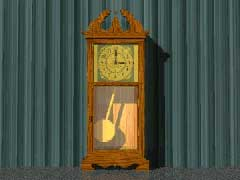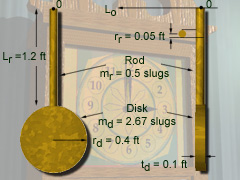Ch 6. Rigid Body Force and Acceleration Multimedia Engineering Dynamics Moment of Inertia Translational Motion Fixed Point Rotation GeneralPlane Motion
 Chapter - Particle - 1. General Motion 2. Force & Accel. 3. Energy 4. Momentum - Rigid Body - 5. General Motion 6. Force & Accel. 7. Energy 8. Momentum 9. 3-D Motion 10. Vibrations Appendix Basic Math Units Basic Equations Sections Search eBooks Dynamics Fluids Math Mechanics Statics Thermodynamics Author(s): Kurt Gramoll ©Kurt GramollDYNAMICS - CASE STUDY IntroductionProblem Graphic Justin Time Clocks Inc. manufactures grandfather clocks with swinging pendulums. To make clocks that keep accurate time, the engineers must determine the mass moment of inertia of the pendulum. What is known: The pendulum pivots about the top at O. The pendulum consists of a slender rod with a disk attached to the end. The rod has a length Lr = 1.2 ft, a mass mr = 0.5 slugs, and its cross section is circular with a radius rr = 0.05 ft. The disk has a thickness td = 0.1 ft, a radius rd = 0.4 ft, and mass md = 2.67 slugs. The rod and disk are made from a homogeneous material with density r.Problem Dimensions Question What is the moment of inertia of the pendulum about an axis Lo passing through its pivot point 0 at the top of the rod? Approach Determine the moment of inertia of each part about an axis passing through each part's center of mass. Determine the moment of inertia of each part about an axis passing through O. Combine the moment of inertia of each part to determine the total moment of inertia of the pendulum about an axis passing through the point O.

Practice Homework and Test problems now available in the 'Eng Dynamics' mobile app
Includes over 400 problems with complete detailed solutions.
Available now at the Google Play Store and Apple App Store.